# alphaShape

Polygons and polyhedra from points in 2-D and 3-D

## Description

An `alphaShape` creates a bounding area or volume that envelops a set of 2-D or 3-D points. You can manipulate the `alphaShape` object to tighten or loosen the fit around the points to create a nonconvex region. You also can add or remove points or suppress holes or regions.

After you create an `alphaShape` object, you can perform geometric queries. For example, you can determine if a point is inside the shape or you can find the number of regions that make up the shape. You also can calculate useful quantities like area, perimeter, surface area, or volume, and plot the shape for visual inspection.

## Creation

To create an `alphaShape` object, use the `alphaShape` function with input arguments that define the shape's vertices. You also can specify an alpha radius and hole or region thresholds when you create the `alphaShape`.

### Syntax

``shp = alphaShape(x,y)``
``shp = alphaShape(x,y,z)``
``shp = alphaShape(P)``
``shp = alphaShape(___,a)``
``shp = alphaShape(___,Name,Value)``

### Description

example

````shp = alphaShape(x,y)` creates a 2-D alpha shape of the points `(x,y)` using the default alpha radius. The default alpha radius produces the tightest fitting alpha shape, which encloses all of the points.`shp` represents a polygon. The polygon has no isolated points or edges, nor does it have dangling edges.```

example

````shp = alphaShape(x,y,z)` creates a 3-D alpha shape of the points `(x,y,z)` using the default alpha radius.`shp` represents a polyhedron. The polyhedron has the previously stated polygon traits, but it additionally does not have isolated faces or dangling faces.```

example

````shp = alphaShape(P)` specifies points `(x,y)` or `(x,y,z)` in the columns of matrix `P`.```

example

````shp = alphaShape(___,a)` creates an alpha shape with alpha radius `a` using any of the arguments in the previous syntaxes.```

example

````shp = alphaShape(___,Name,Value)` uses additional options specified by one or more `Name,Value` pair arguments. For example, you can suppress interior holes or voids using `'HoleThreshold'`.```

### Input Arguments

expand all

x-coordinates of points, specified as a column vector.

Data Types: `double`

y-coordinates of points, specified as a column vector.

Data Types: `double`

z-coordinates of points, specified as a column vector.

Data Types: `double`

Point coordinates, specified as a matrix with two columns (for a 2-D alpha shape) or a matrix with three columns (for a 3-D alpha shape).

• For 2-D, the columns of `P` represent `x` and `y` coordinates, respectively.

• For 3-D, the columns of `P` represent `x`, `y`, and `z` coordinates, respectively.

Data Types: `double`

Alpha radius, specified as a nonnegative scalar. The default alpha radius is `a = criticalAlpha(shp,'all-points')`, which is the smallest alpha radius that produces an alpha shape that encloses all points.

Specify `a = criticalAlpha(shp,'one-region')` to use the smallest alpha radius that produces an alpha shape with only one region.

The extreme values of `a` are

• `Inf`, where `alphaShape` produces the convex hull

• `0`, where `alphaShape` produces an empty alpha shape

Data Types: `double`

Name-Value Pair Arguments

Specify optional comma-separated pairs of `Name,Value` arguments. `Name` is the argument name and `Value` is the corresponding value. `Name` must appear inside quotes. You can specify several name and value pair arguments in any order as `Name1,Value1,...,NameN,ValueN`.

Example: ```shp = alphaShape(...,'HoleThreshold',10)```

Maximum area or volume of interior holes or voids to fill in, specified as a finite, nonnegative scalar.

• For 2-D, `HoleThreshold` specifies the maximum area of interior holes to fill in.

• For 3-D, `HoleThreshold` specifies the maximum volume of interior voids to fill in. Holes extending completely through the alpha shape cannot be filled in.

When you specify both a `'HoleThreshold'` and a `'RegionThreshold'`, the application of the thresholds is order dependent. `alphaShape` fills in holes before suppressing regions.

Data Types: `double`

Maximum area (2-D) or volume (3-D) of regions to suppress, specified as a finite, nonnegative scalar.

When you specify a `'HoleThreshold'` and a `'RegionThreshold'`, the application of the thresholds is order dependent. `alphaShape` fills in holes before suppressing regions.

Data Types: `double`

## Properties

expand all

Coordinates of points, specified as a matrix with two or three columns (for 2-D or 3-D point sets). These points are initially used to create the alpha shape, excluding duplicates.

Data Types: `double`

Alpha radius, specified as a nonnegative scalar. The alpha radius is the radius of the alpha disk or sphere that sweeps over the points to create the alpha shape.

The default alpha radius is ```a = criticalAlpha(shp,'all-points')```, which is the smallest alpha radius that produces an alpha shape enclosing all points. Specify ```a = criticalAlpha(shp,'one-region')``` to use the smallest alpha radius that produces an alpha shape with only one region.

The extreme values of `Alpha` have the following conditions:

• If `Alpha` is `Inf`, then `alphaShape` produces the convex hull.

• If `Alpha` is `0`, then the resulting `alphaShape` is empty.

Data Types: `double`

Maximum area or volume of interior holes or voids to fill in, specified as a finite nonnegative scalar.

• For 2-D, `HoleThreshold` specifies the maximum area of interior holes to fill in.

• For 3-D, `HoleThreshold` specifies the maximum volume of interior voids to fill in. Holes extending completely through the 3-D alpha shape cannot be filled in.

The default value is `0`, so that `alphaShape` does not suppress any holes or voids. The application of the `HoleThreshold` and `RegionThreshold` properties is order-dependent. `alphaShape` fills in holes before suppressing regions.

Data Types: `double`

Maximum area (2-D) or volume (3-D) of regions to suppress, specified as a finite nonnegative scalar.

The default value is `0`, so that `alphaShape` does not suppress any regions. The application of the `HoleThreshold` and `RegionThreshold` properties is order-dependent. `alphaShape` fills in holes before suppressing regions.

Data Types: `double`

## Object Functions

 `alphaSpectrum` Alpha values giving distinct alpha shapes `criticalAlpha` Alpha radius defining critical transition in shape `numRegions` Number of regions in alpha shape `inShape` Determine if point is inside alpha shape `alphaTriangulation` Triangulation that fills alpha shape `boundaryFacets` Boundary facets of alpha shape `perimeter` Perimeter of 2-D alpha shape `area` Area of 2-D alpha shape `surfaceArea` Surface area of 3-D alpha shape `volume` Volume of 3-D alpha shape `plot` Plot alpha shape `nearestNeighbor` Determine nearest alpha shape boundary point

## Examples

collapse all

Find the shape of a 2-D point cloud of data.

Create and plot a set of 2-D points.

```th = (pi/12:pi/12:2*pi)'; x1 = [reshape(cos(th)*(1:5), numel(cos(th)*(1:5)),1); 0]; y1 = [reshape(sin(th)*(1:5), numel(sin(th)*(1:5)),1); 0]; x = [x1; x1+15]; y = [y1; y1]; plot(x,y,'.') axis equal```Compute an alpha shape for the point set using the default alpha radius.

```shp = alphaShape(x,y); plot(shp)```Check the value of the default alpha radius.

`shp.Alpha`
```ans = 0.7752 ```

The default alpha radius results in an alpha shape with a jagged boundary. To better capture the boundary of the point set, try a larger alpha radius.

Compute an alpha shape using an alpha value of 2.5.

```shp.Alpha = 2.5; plot(shp)```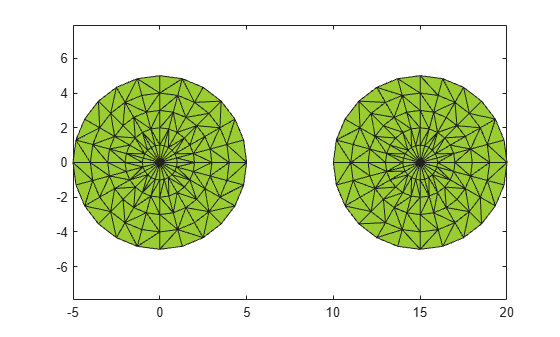Find the shape of a 3-D point cloud of data.

Create and plot a set of 3-D points.

```[x1,y1,z1] = sphere(24); x1 = x1(:); y1 = y1(:); z1 = z1(:); x2 = x1+5; P = [x1 y1 z1; x2 y1 z1]; P = unique(P,'rows'); plot3(P(:,1),P(:,2),P(:,3),'.') axis equal grid on```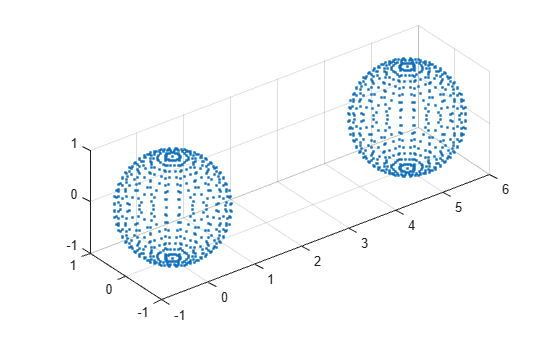Compute a 3-D alpha shape using an alpha radius of 1.

```shp = alphaShape(P(:,1),P(:,2),P(:,3),1); plot(shp) axis equal```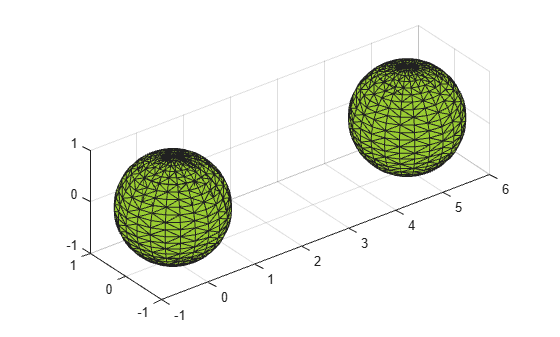Create an alpha shape by specifying its alpha radius, and fill the holes in an alpha shape.

Create and plot a 2-D set of points.

```th = (pi/12:pi/12:2*pi)'; x1 = [reshape(cos(th)*(2:5), numel(cos(th)*(2:5)),1);]; y1 = [reshape(sin(th)*(2:5), numel(sin(th)*(2:5)),1);]; x = [x1; x1+15;]; y = [y1; y1]; plot(x,y,'.') axis equal```Compute an alpha shape for the point set using an alpha radius of 1.

```shp = alphaShape(x,y,1); plot(shp)```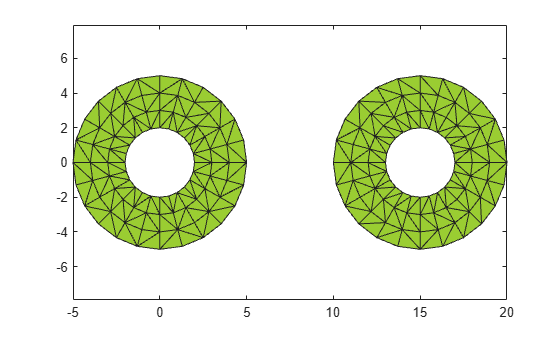An alpha radius of 1 results in an alpha shape with two regions containing holes. To suppress the small holes in the alpha shape, you can specify a `HoleThreshold` by estimating the area of the largest hole to fill. To fill all holes in the shape, you can assign an arbitrarily large value to `HoleThreshold`.

Create a new alpha shape that suppresses the holes by specifying a `HoleThreshold` of 15.

```shp = alphaShape(x,y,1,'HoleThreshold',15); plot(shp)```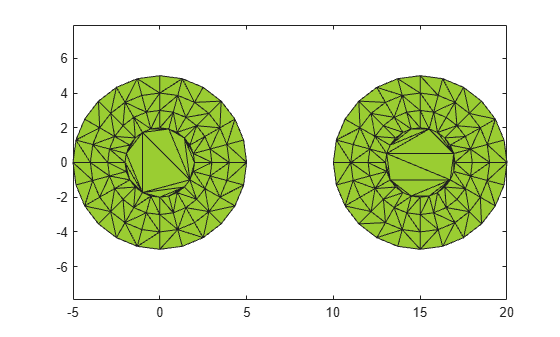Control the number of regions of an alpha shape by setting a region threshold.

Create and plot a set of 3-D points.

```[x1,y1,z1] = sphere(24); x1 = x1(:); y1 = y1(:); z1 = z1(:); x2 = x1+5; [x3,y3,z3] = sphere(5); x3 = x3(:)+5; y3 = y3(:); z3 = z3(:)+25; P = [x1 y1 z1; x2 y1 z1; 0.25*x3 0.25*y3 0.25*z3]; P = unique(P,'rows'); plot3(P(:,1),P(:,2),P(:,3),'.') axis equal grid on```Compute an alpha shape for the point set using an alpha radius of 1.

```shp = alphaShape(P,1); plot(shp) axis equal```In this case, the alpha shape produces a small region above the two equal-sized spheres. To suppress this region, you can specify a `RegionThreshold` by estimating its volume.

Specify a `RegionThreshold` of 2. The resulting shape contains only the two larger regions.

```shp.RegionThreshold = 2; plot(shp) axis equal```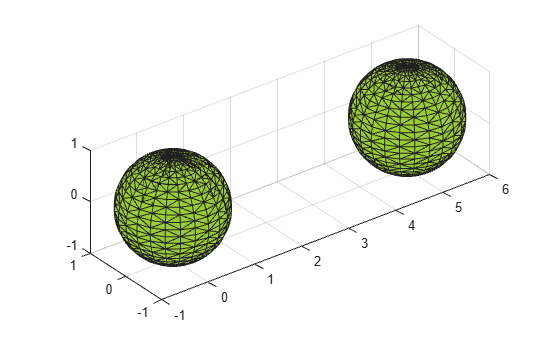Add points to an existing alpha shape.

Create and plot a 2-D set of points.

```th = (pi/12:pi/12:2*pi)'; x1 = [reshape(cos(th)*(1:5), numel(cos(th)*(1:5)),1); 0]; y1 = [reshape(sin(th)*(1:5), numel(sin(th)*(1:5)),1); 0]; x = [x1; x1+15;]; y = [y1; y1]; plot(x,y,'.') axis equal```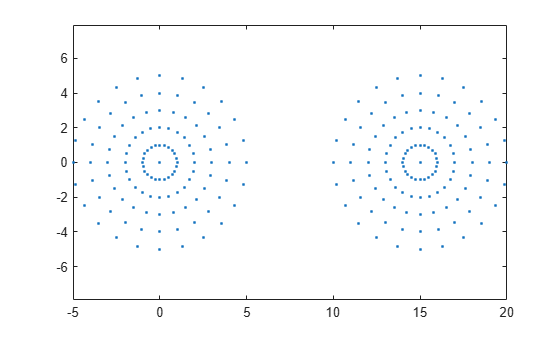Compute an alpha shape for the point set using an alpha radius of 1. The resulting alpha shape has two regions.

```shp = alphaShape(x,y,1); plot(shp)```Now add a third region to the alpha shape by adding new points directly to the `shp.Points` matrix.

```x3 = x1+8; y3 = y1+10; shp.Points(end+1,:) = [x3 y3]; plot(shp)```